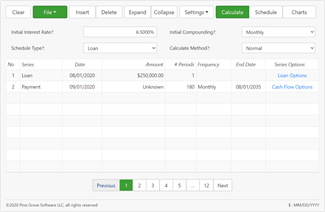##### Ultimate Financial Calculator™

Time-value-of-money calculations with regular or irregular cash flows. Solve for:

• Present Value (PV)
• Future Value (FV)
• Payment amount, rate or term
• Exact loan payoff amount
• 25 step-by-step tutorials

The Ultimate Financial Calculator (UFC) is the most sophisticated, most flexible calculator on financial-calculators.com and I think on the entire internet. It works extraordinarily well as both a time value of money calculator and as a loan or mortgage payoff calculator.

See the tutorials below for step-by-step instructions.

If you are someone who needs date accurate results with either regular or irregular cash flows (loans, payments, deposits, withdrawals, investments), this is the calculator you should study and use.

Questions?

For best results, turn your device
Idx No Series Date Amount # Periods Frequency End Date Series Options cmpFreq specialSeriesType SpecialSeriesStruct
×

#### Info...

Original Size

## What is Time Value of Money?

Time Value of Money (TVM) is the concept that the value of money itself changes over time. Having a dollar today is worth more than a dollar tomorrow. Solving for present value, future value, amount, interest rate and term are some standard time value of money (Wikipedia) calculations. The UFC is capable of performing any of these calculations with regular or irregular amounts as of any date for investment, savings or loan cash flow.

### Time value of money example

As a time value of money calculator, UFC can calculate unknown amounts for complex and irregular cash flows. The below example answers the question, "How much do I need to invest for 48 quarterly periods to have a total future value that will then let me withdrawal \$1,000 monthly for 180 months?"

To answer this question, set the calculator up as shown:

If you are someone who regularly needs to do TVM calculations, then it is worth your time to study the features this calculator offers. It can replace easily three-quarters of the calculators found on this site — and on other websites too!

## Calculator's Features

### Solve for any unknown

• Payment or loan amount
• Deposit or withdrawal
• Yields: APR, APY or IRR
• Balance as of a specific date
• Date a specific balance is reached *
• Present value (PV)
• Future value (FV)
• Balloon payment amount
• Payment required to reach a specific balloon
• Number of payments
• Interest rates - nominal or effective *
• Discounted values
• Remaining balance
• Deposit required

### Any type of calculation method

• Normal amortization or investment
• Rule-of-78s
• US Rule — simple interest
• Supports 360, 364 and 365 day years
• Exact day or periodic interest calculations

### Scheduled (but adjustable) Payment Frequencies

• Daily
• Weekly
• Bi-weekly
• Twice monthly (Half-month)
• Every 4 weeks
• Monthly
• Bi-monthly (every two months)
• Quarterly
• Every 4 months
• Semi-annual
• Annual

* Feature only available in C-Value! ™,
our cash flow calculator for Windows™

### Flexible Reports & Schedules

• Amortization & investment schedules
• Schedules with details or totals only *
• Custom headers and labels *
• Change fonts, colors *
• Select a fiscal year end *
• Reg. Z APR disclosure report *

### Handles any type of cash flow

• Normal
• Interest only
• Enter your own payment amount
• Negative amortization
• Skipped payments or deposits
• Fixed principal + interest
• Percent step amounts
• Dollar step amounts
• Balloon payments
• Extra payments — principal only
• Payments to interest
• Cash flow amounts set to any random date

### Compounding Frequencies

• Exact day simple
• Daily compounding
• Weekly
• Bi-weekly
• Twice monthly (Half-month)
• Every 4 weeks
• Monthly
• Bi-monthly (every two months)
• Quarterly
• Every 4 months
• Semi-annual
• Annual
• Continuous
• Change the frequency of compounding during a cash flow
• No compounding option when rate changes

## Using UFC as a Mortgage or Loan Payoff Calculator

A well-designed loan payoff calculator will answer any of these questions:

• How many payments do I have left?
• When will my loan be paid off if I make extra payments?
• What payment is required to pay a loan off by a given date?
• The borrower missed payments, paid late and paid additional amounts and there were interest rate changes, what is the exact loan balance due as of today?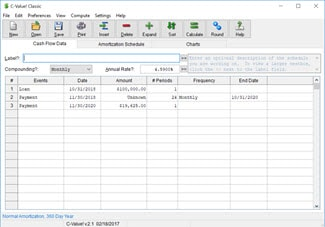##### C-Value!, A TVM Calculator for Windows

An extremely flexible time-value-of-money calculator for Windows computers.

• Loan or investment calculations.
• Cash flows can be regular or irregular
• Create and print schedules.
• Save your data to disk for later use.

Suitable for auditors, accountants, lawyers and you!

Answering the first three questions is straightforward and takes but a second, but, as you may have guessed, calculating the payoff amount for the fourth scenario is more involved. For instructions on how to use the UFC as a tool for tracking a mortgage or loan balance with payment and interest rate changes, read my tutorial Calculate Loan Balance — Loan Payoff Calculation.

### Mortgage or loan payoff examples

If you want to know how many payments are left or when the last payment is due, enter the current interest rate (4% for our example) and set compounding. Then in row one, enter the last known loan balance and the balance as of date (\$250,000 and Sept. 1). In the 2nd row enter the due date of the next payment after the loan balance date in row one (this may also be the balance date), enter the scheduled payment amount, set "#Periods" to "Unknown" and set the payment frequency (monthly). Your screen will look like this:

After clicking "Calculate," your screen should look like below. There are 143 remaining payments, and the last payment will be due on August 1.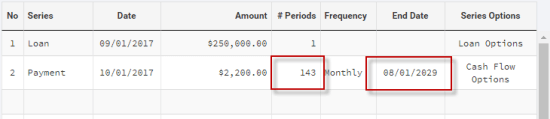Loan payoff result showing payments remaining and due date of the last payment.

Now, let's delve in a bit deeper. You plan to pay an extra \$150 a month on your mortgage, and you want to know the payoff date. The UFC excels as an early payoff calculator. If we use the above example, all you need to do is change the payment amount to \$2,350.00 and set "#Periods" to "Unknown" again.

Click "Calculate" once again. Your screen should look like below. Now only 132 payments are remaining, and the last payment will be due on September 1 a year earlier.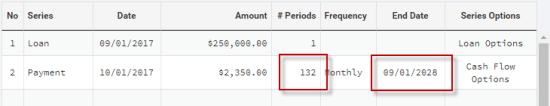Early payoff result showing payments remaining and due date of the last payment.

Now, if you're lucky, the mortgage is paid off just as the first child is going off to college. :-)

## Financial Calculations Step-by-Step Tutorials

The below tutorials walk you through the steps for setting up the indicated financial calculation. I recommend that you right click on a link and select "Open in New Window" so you can have the calculator handy in this window as you read.

1. Calculate Payment
• loan or mortgage periodic payment calculation
• also an introduction to this calculator
2. Investment Cash Flow
• calculating final value
3. Calculate Income From An Investment
• How to calculate income you can expect from an investment
4. Adjustable Rate Mortgage or Loan Calculator
• ARM with interest rate changes on any date you desire
5. Calculate a Loan's Term
• How to solve for an unknown number of payments
6. Calculate Loan Amount
• How much can I borrow?
7. Balloon Payment Calculation
• Calculate the balloon amount
8. Balloon Loan Calculation
• Calculate the periodic payment required to result in a specified balloon
9. Random Extra Principal Payment
• How to prepay principal on any date
10. Loan with Series of Extra Principal Payments
• How to calculate loan or mortgage with extra payments
11. Construction Loan Calculator
• Generally a short term loan with multiple borrows
12. Monthly Skipped Payments
• Loan or mortgage with scheduled skipped payments
13. Odd Length First Period
• Interest payment options for initial period
14. Interest Only Loan
• Initial series of interest only payments
15. Biweekly Mortgage Payments
• Pay 1/2 the monthly payment every other week to reduce the total interest paid
16. US Rule
• No interest charged on interest — separate tracking of interest balance
17. How much do I have to save or invest?
• State your goal - calculate periodic investment amount needed to reach goal
18. Paying for College
• You may have longer than you think
• Multiple investments with multiple, overlapping withdrawals
• Demonstrates solving for unknown in complex cash flow
19. Future Value Calculation
• How to set up simple or complex cash flows to calculate FV
20. Present Value Calculation
• How to discount a simple or complex cash flow to find its PV
21. Calculate PV of Fixed Principal + Interest Loan
• Calculate PV of the declining payment amount
• Demonstrates the cash flow analytics of this calculator
22. Calculate Rate of Return (ROR) on an Annuity
• How to set up an annualized ROR calculation
23. Calculate Time It Takes to Reach Investment Goal
• Set a goal and see how long it takes to reach it
24. Calculate ROI for X Days
• Exact day return on investment calculation
25. Calculate Loan Balance — Loan Payoff Calculation
• Enter payments for any amount on date made — audit balance due

## Calculators the Ultimate Financial Calculator Replaces

With this calculator's flexibility, it will meet the needs of anyone searching for:

• loan repayment calculator
• loan payoff calculator
• mortgage payoff calculator
• repayment calculator
• student loan repayment calculator
• home loan repayment calculator
• car loan repayment calculator
• debt payoff calculator
• early mortgage payoff calculator
• debt repayment calculator
• individual or specialty TVM calculators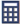Join the conversation. Tell me what you think.